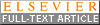# DEcomposition and Component Analysis of Exponential Signals (DECAES)

## Introduction

DECAES provides tools for decomposing multi-exponential signals which arise from multi spin-echo magnetic resonance imaging (MRI) scans into exponential components. The main decomposition method used is an inverse Laplace transform-based technique which involves solving the regularized nonnegative least squares (NNLS) inverse problem

$$$X = \mathrm{argmin}_{x \ge 0} ||Ax - b||_2^2 + \mu^2 ||x||_2^2$$$

where $b$ is the signal magnitude data, $A$ is a matrix of exponential decay bases, and $\mu$ is a regularization parameter. $A$ is constructed using the extended phase graph algorithm with stimulated echo correction. The columns of $A$ are exponential bases with differing characteristic $T_2$ decay times $T_{2,j}$.

The output $X$ is the spectrum of (nonnegative) exponential decay amplitudes. Amplitude $X_j$ of the spectrum $X$ is therefore interpreted physically as the amount of the signal $b$ which decays with time constant $T_{2,j}$. For this reason, the spectrum $X$ is commonly referred to as the $T_2$ distribution. DECAES provides methods for computing $T_2$-distributions.

## Installation

Start julia from the command line, type ] to enter the package manager REPL mode (the julia> prompt will be replaced by a pkg> prompt), and enter the following command:

pkg> add DECAES

Once the package is finished installing, type the backspace key to exit the package manager REPL mode (the julia> prompt should reappear). Exit Julia using the keyboard shortcut Ctrl+D, or by typing exit().

## Updating DECAES

To update DECAES to the latest version, start julia from the command line, type ] to enter the package manager REPL mode, and enter the following:

pkg> update DECAES

## Myelin water imaging

Myelin water imaging (MWI) is an MRI technique used to visualize the myelin water contained within the sheaths of myelinated axons within the body, such as within the brain's white matter.

Through analysing $T_2$-distributions computed from multi spin-echo MRI scans, one can separate the contribution due to the myelin water from the intra- and extra-cellular water and compute the myelin water fraction (MWF). The MWF describes the fraction of water trapped between myelin lipid bilayers relative to the total water in the region. DECAES provides methods for computing the MWF.

MWI was pioneered at the University of British Columbia by Alex MacKay and Ken Whittal.

Basics of myelin water imaging:

Validation of myelin water imaging:

Some applications of myelin water imaging:

## Acknowledgements

• Porting to Julia was done by Jonathan Doucette (email: jdoucette@physics.ubc.ca) in November 2019. This work was funded by NSERC (016-05371) and CIHR (RN382474-418628) under PI Alexander Rauscher at the University of British Columbia
• Christian Kames (email: ckames@physics.ubc.ca) contributed to optimizing the Julia port for both speed and memory efficiency, as well as writing the PAR/XML/REC file reader used internally
• Original MATLAB code was written by Thomas Prasloski (email: tprasloski@gmail.com). Modifications to the MATLAB code were made by Vanessa Wiggermann to enable processing on various MATLAB versions in February 2019. The Julia port is based on this modified version

## Citing this work@article{DECAES.jl-2020,
}